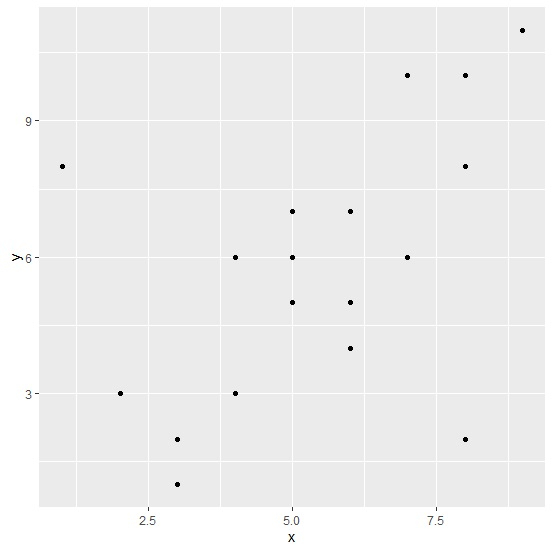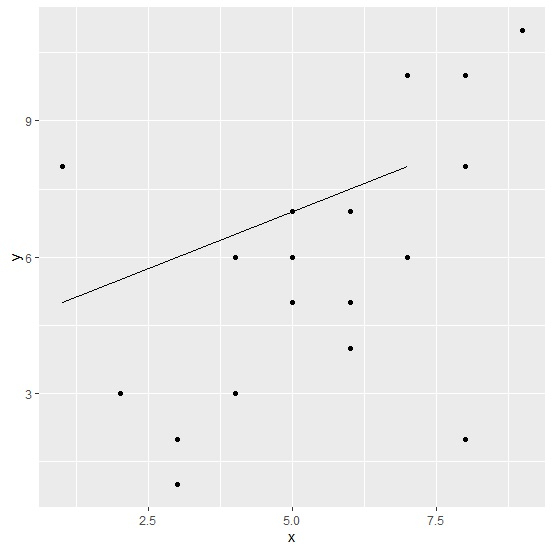# How to display a line in segment of a plot using ggplot2 in R?

To display a line in segment of a plot, we can use geom_segment function of ggplot2 package where we need to pass the initial and the ending values for both the axes. For example, if we have data frame called df that contains x and y then a scatterplot with a line segment can be created by using the below command −

ggplot(df,aes(x,y))+geom_point()+
geom_segment(aes(x=xstart,xend=xlast,y=ystart,yend=ylast))

Consider the below data frame −

## Example

Live Demo

x<-rpois(20,5)
y<-rpois(20,5)
df<-data.frame(x,y)
df

## Output

   x y
1  4 6
2  6 4
3  8 10
4  5 7
5  8 2
6  2 3
7  7 6
8  1 8
9  4 3
10 5 5
11 6 5
12 3 1
13 9 11
14 6 7
15 7 10
16 8 8
17 5 6
18 5 5
19 3 2
20 5 5

Loading ggplot2 package and creating a scatterplot of x and y −

## Example

library(ggplot2)
ggplot(df,aes(x,y))+geom_point()

## OutputCreating a scatterplot of x and y with a line segment −

## Example

ggplot(df,aes(x,y))+geom_point()+geom_segment(aes(x=1,xend=7,y=5,yend=8))

## Output